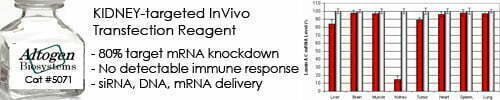••••Shop Products

# Actual Yield Calculator

An actual yield calculator is a tool that calculates the actual yield of a chemical reaction based on the amount of product obtained in the reaction and the theoretical yield of the reaction. The actual yield is the amount of product that is actually obtained in a chemical reaction, while the theoretical yield is the amount of product that would be obtained if the reaction proceeded perfectly without any losses.

The formula for calculating actual yield as a percentage of theoretical yield is:

Actual Yield % = (Actual Yield / Theoretical Yield) x 100%

Where:

• Actual Yield is the amount of product actually obtained in the reaction
• Theoretical Yield is the maximum amount of product that could be obtained based on stoichiometry calculations and assuming complete conversion of all reactants to products

To use an actual yield calculator, you need to input the values of actual yield and theoretical yield, and the calculator will calculate the corresponding percentage yield for you. The resulting percentage yield value can then be used to assess the efficiency of the chemical reaction and to identify potential sources of error or losses in the reaction.

Actual yield is an important concept in chemistry, where it is used to assess the efficiency of chemical reactions, optimize reaction conditions, and calculate reaction kinetics and thermodynamics.

Actual Yield Calculator
Theoretical Yield:
g
Percent Yield:
%

Sorry, comments are closed for this post.